1. 数组

2021/5/28 14:03:10 人评论

数组

3. 访问(Access)和搜索(Search)

**访问 **是 通过下标是找这个元素的值

**搜索 **是 直接查找元素

int a[1,2,3]

6. 常用操作

1. 创建数组

1. int[] arr = {1,2,3};
2. int[] arr = new int[]{1,2,3};

1. int[] arr = new int; 当不知道数组应该放多少个元素，用这个
2. ArrayList<Integer> arr =new ArrayList<>(); 不需要指定长度和元素，用这个

2. 添加元素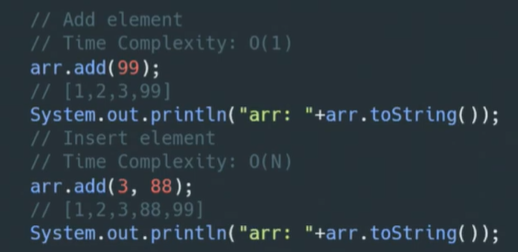O(1)是插在尾端，O(N)是插在中间

3. 访问元素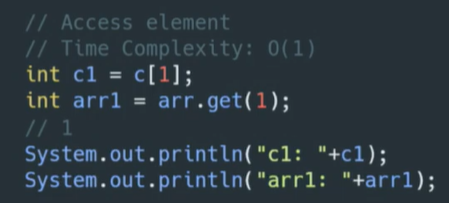c是普通方法，

arr.get(1)是ArrayList的方法

4. 修改（更新）元素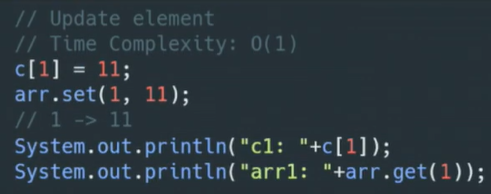c是普通方法，

arr.set(1,11)是ArrayList的方法

5. 删除元素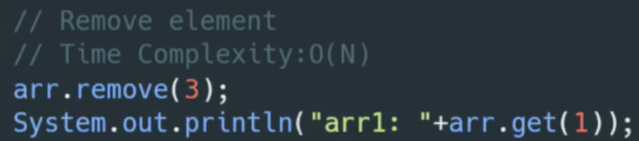6. 数组的长度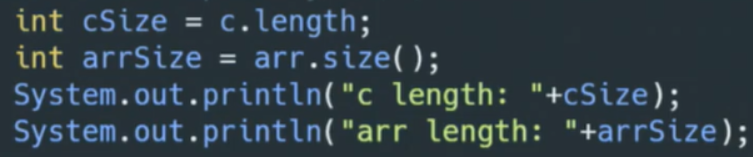c.length是普通方法，

arr.size()是ArrayList的方法

7. 遍历元素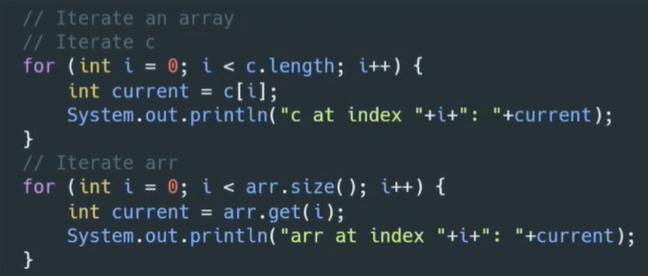c.length是普通方法，

arr.size()是ArrayList的方法

8. 查找元素ArrayList方式，用contains()方法

9. 数组排序(内置排序法)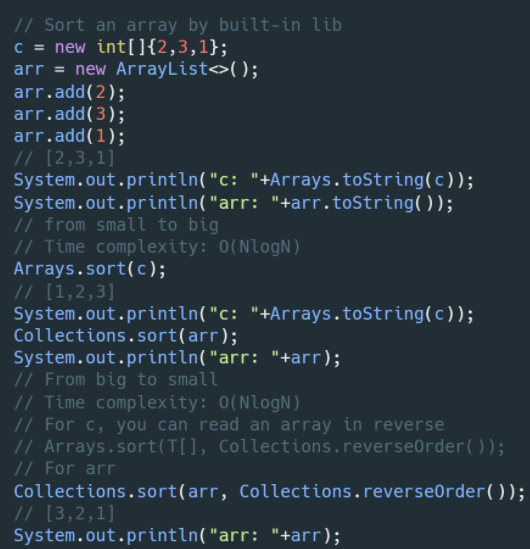​ 普通：Arrays.sort(T[],Collections.reverseOrde()); 此题中，T[]Integer[] c,要传对象方式

​ ArrayList:Collections.sort(arr, Collections.reverseOrder( ));

Leetcode

485.最大连续1的个数

1.先判断数组长度是否为0或者数组为空

2.定义一个计数器count，和存放结果的result

3.遍历数组，如果元素为1，计数器+1

4.遍历完毕后，返回=result和count的最大值

public class array {
public int findMaxConsecutiveOnes(int[] nums) {
if (nums.length == 0 || nums == null) {
return 0;
}
int count = 0;
int result = 0;
for (int i = 0; i < nums.length; i++) {
if (nums[i] == 1) {
count++;
} else {
result = Math.max(result, count);
count = 0;
}
}
return Math.max(result, count);
}
}

283.移动零

public class zero {
public void moveZeroes(int[] nums) {
int index = 0;
for (int i = 0; i < nums.length; i++) {
if (nums[i] != 0) {
nums[index] = nums[i];
index++;
}
}
for (int i = index; i < nums.length; i++) {
nums[i] = 0;
}
}

package qi.array;

/**
* 移动0
*/
public class zero {
public void moveZeroes(int[] nums) {
if(nums==null) {
return;
}
//两个指针i和j
int j = 0;
for(int i=0;i<nums.length;i++) {
//当前元素!=0，就把其交换到左边，等于0的交换到右边
if(nums[i]!=0) {
int tmp = nums[i];
nums[i] = nums[j];
nums[j++] = tmp;
}
}

}

27.移除元素

1. 判断

2. 定义两个指针

3. 在 左< 右 的时候

如果左 != 值，左指针右移，

如果 右 = 值，右指针左移

4. 交换左右指针上的的元素

5. 返回，如果 左指针或者右指针（因为最后两个指针会指向同一个位置） 上的元素等于val，返回当前指针，否则返回指针加一

public int removeElement(int[] nums, int val) {
if (nums == null || nums.length == 0) {
return 0;
}
int l = 0;
int r = nums.length - 1;
while (l < r) {
while (l < r && nums[l] != val) {
l++;
}
while (l < r && nums[r] == val) {
r--;
}
int tmp = nums[l];
nums[l] = nums[r];
nums[r] = tmp;

}

return nums[l] == val ? l : l + 1;
}

public int removeElement(int[] nums, int val) {
if (nums == null || nums.length == 0) {
return 0;
}
int j = 0;
for (int i = 0; i < nums.length; i++) {
if (nums[i] != val) {
nums[j] = nums[i];
j++;
}
}
return j;
}

public int removeElement(int[] nums, int val) {
int size = nums.length;
for (int i = 0; i < size; i++) {
if (nums[i] == val) { // 发现需要移除的元素，就将数组集体向前移动一位
for (int j = i + 1; j < size; j++) {
nums[j - 1] = nums[j];
}
i--; // 因为下表i以后的数值都向前移动了一位，所以i也向前移动一位
size--; // 此时数组的大小-1
}
}
return size;
}

暂无相关的数据...